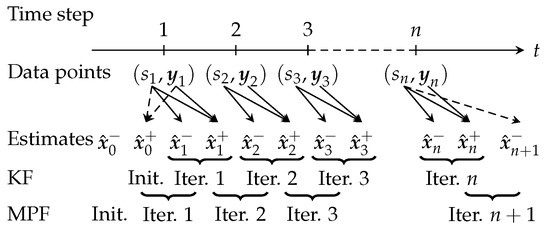Next Article in Journal
A Hierarchical Approach for Joint Parameter and State Estimation of a Bilinear System with Autoregressive Noise
Next Article in Special Issue
The Averaged Hausdorff Distances in Multi-Objective Optimization: A Review
Open AccessArticle

# An Iterative Method Based on the Marginalized Particle Filter for Nonlinear B-Spline Data Approximation and Trajectory Optimization

byJens Jauch,Felix Bleimund,Michael Frey *andFrank Gauterin
Institute of Vehicle System Technology, Karlsruhe Institute of Technology, 76131 Karlsruhe, Germany
*
Author to whom correspondence should be addressed.
Mathematics 2019, 7(4), 355; https://doi.org/10.3390/math7040355
Received: 3 February 2019 / Revised: 1 April 2019 / Accepted: 10 April 2019 / Published: 16 April 2019
(This article belongs to the Special Issue Recent Trends in Multiobjective Optimization and Optimal Control)
The B-spline function representation is commonly used for data approximation and trajectory definition, but filter-based methods for nonlinear weighted least squares (NWLS) approximation are restricted to a bounded definition range. We present an algorithm termed nonlinear recursive B-spline approximation (NRBA) for an iterative NWLS approximation of an unbounded set of data points by a B-spline function. NRBA is based on a marginalized particle filter (MPF), in which a Kalman filter (KF) solves the linear subproblem optimally while a particle filter (PF) deals with nonlinear approximation goals. NRBA can adjust the bounded definition range of the approximating B-spline function during run-time such that, regardless of the initially chosen definition range, all data points can be processed. In numerical experiments, NRBA achieves approximation results close to those of the Levenberg–Marquardt algorithm. An NWLS approximation problem is a nonlinear optimization problem. The direct trajectory optimization approach also leads to a nonlinear problem. The computational effort of most solution methods grows exponentially with the trajectory length. We demonstrate how NRBA can be applied for a multiobjective trajectory optimization for a battery electric vehicle in order to determine an energy-efficient velocity trajectory. With NRBA, the effort increases only linearly with the processed data points and the trajectory length. View Full-Text
Show FiguresFigure 1

MDPI and ACS Style

Jauch, J.; Bleimund, F.; Frey, M.; Gauterin, F. An Iterative Method Based on the Marginalized Particle Filter for Nonlinear B-Spline Data Approximation and Trajectory Optimization. Mathematics 2019, 7, 355.